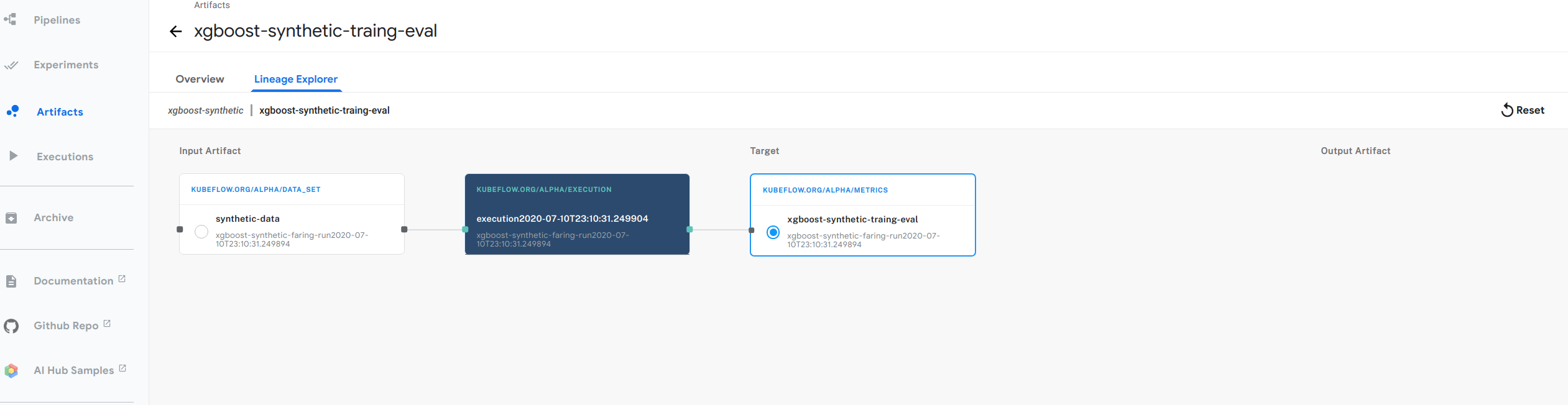# Lineage Tracking

• This blog post will first guide you through the metadata SDK API, to create a notebook and log several actions to the metadata DB. Afterwards, you will be able to navigate to the Kubeflow UI and the resulting lineage graph, which gives you a graphical representation of the dependencies between the objects you logged using the SDK.

```!pip install kubeflow-metadata --user
# Install other packages:
!pip install pandas --user
# Then restart the Notebook kernel.
```
```import pandas
from datetime import datetime
from uuid import uuid4
```
```METADATA_STORE_HOST = "metadata-grpc-service.kubeflow" # default DNS of Kubeflow Metadata gRPC serivce.
```

## Create a new Workspace and Run in a workspace

• A Workspace groups a set of pipelines or notebooks runs, and their related artifacts and executions
• Store is an object that provides a connection to the Metadata gRPC service
• The Run object captures a pipeline or notebook run in a workspace
```ws1 = metadata.Workspace(
# Connect to metadata service in namespace kubeflow in k8s cluster.
name="xgboost-synthetic",
description="workspace for xgboost-synthetic artifacts and executions",
labels={"n1": "v1"})
```
```r = metadata.Run(
workspace=ws1,
name="xgboost-synthetic-faring-run" + datetime.utcnow().isoformat("T") ,
description="a notebook run",
)
```

## Create an execution in a run

• An Execution is a specific instance of a run, and you can bind specific input/output artifacts to this instance. Execution also serves as object for logging artifacts as its input or output
```exec = metadata.Execution(
name = "execution" + datetime.utcnow().isoformat("T") ,
workspace=ws1,
run=r,
description="execution for training xgboost-synthetic",
)
print("An execution was created with id %s" % exec.id)
```
```An execution was created with id 290
```

## Log a data set and a model

• A Log_input log an artifact as an input of this execution. Here exec.log_input accept an artifact class as an argument, a DataSet is an artifact. Every artifacts has different paramenters such as name, uri, query. The way to create DataSet artifact is calling ready-to-use APIs metadata.DataSet and provide arguments
```date_set_version = "data_set_version_" + str(uuid4())
data_set = exec.log_input(
description="xgboost synthetic data",
name="synthetic-data",
owner="someone@kubeflow.org",
uri="file://path/to/dataset",
version="v1.0.0",
query="SELECT * FROM mytable"))
print("Data set id is {0.id} with version '{0.version}'".format(data_set))
```
```Data set id is 171 with version 'data_set_version_cbebc757-0d76-4e1e-bbd9-02b065e4c3ea'
```
• A Log_output log an artifact as a output of this execution. Here exec.log_output accept an artifact class as an argument, a Model is an artifact. Every artifacts has different paramenters such as name, uri, hyperparameters. The way to create Model artifact is calling ready-to-use APIs metadata.Model and provide arguments
```model_version = "model_version_" + str(uuid4())
model = exec.log_output(
name="MNIST",
description="model to recognize handwritten digits",
owner="someone@kubeflow.org",
uri="gcs://my-bucket/mnist",
model_type="neural network",
training_framework={
"name": "tensorflow",
"version": "v1.0"
},
hyperparameters={
"learning_rate": 0.5,
"layers": [10, 3, 1],
"early_stop": True
},
version=model_version,
labels={"mylabel": "l1"}))
print(model)
print("\nModel id is {0.id} and version is {0.version}".format(model))
```
```kubeflow.metadata.metadata.Model(workspace=None, name='MNIST', description='model to recognize handwritten digits', owner='someone@kubeflow.org', uri='gcs://my-bucket/mnist', version='model_version_50b419e2-af69-4c0e-a251-78246d4c0578', model_type='neural network', training_framework={'name': 'tensorflow', 'version': 'v1.0'}, hyperparameters={'learning_rate': 0.5, 'layers': [10, 3, 1], 'early_stop': True}, labels={'mylabel': 'l1'}, id=172, create_time='2019-12-04T00:44:49.444411Z', kwargs={})

Model id is 172 and version is model_version_50b419e2-af69-4c0e-a251-78246d4c0578
```

## Log the evaluation of a model

• Metrics captures an evaluation metrics of a model on a data set
```metrics = exec.log_output(
name="MNIST-evaluation",
description="validating the MNIST model to recognize handwritten digits",
owner="someone@kubeflow.org",
uri="gcs://my-bucket/mnist-eval.csv",
data_set_id=str(data_set.id),
model_id=str(model.id),
values={"accuracy": 0.95},
labels={"mylabel": "l1"}))
print("Metrics id is %s" % metrics.id)
```
```Metrics id is 173
```

```serving_application = metadata.Execution(
name="serving model",
workspace=ws1,
description="an execution to represent model serving component",
)
# Noticed we use model name, version, uri to uniquely identify existing model.
name="MNIST",
uri="gcs://my-bucket/mnist",
version=model.version,
)
m=serving_application.log_input(served_model)
print("Found the mode with id {0.id} and version '{0.version}'.".format(m))
```
```Found the mode with id 172 and version 'model_version_50b419e2-af69-4c0e-a251-78246d4c0578'.
```

# Plot the lineage graph• The figure above shows an example of the lineage graph from our xgboost example. Follow below steps for you to try out:
1. Follow the guide to setting up your Jupyter notebooks in Kubeflow
2. Go back to your Jupyter notebook server in the Kubeflow UI. (If you’ve moved away from the notebooks section in Kubeflow, click Notebook Servers in the left-hand navigation panel to get back there.)
3. In the Jupyter notebook UI, click Upload and follow the prompts to upload the xgboost example notebook.
4. Click the notebook name (build-train-deploy.ipynb.ipynb) to open the notebook in your Kubeflow cluster.
5. Run the steps in the notebook to install and use the Metadata SDK.
6. Click Artifact Store in the left-hand navigation panel on the Kubeflow UI.
7. Select Pipelines -> Artifacts
8. Navigate to xgboost-synthetic-traing-eval
9. Click on Lineage explorer